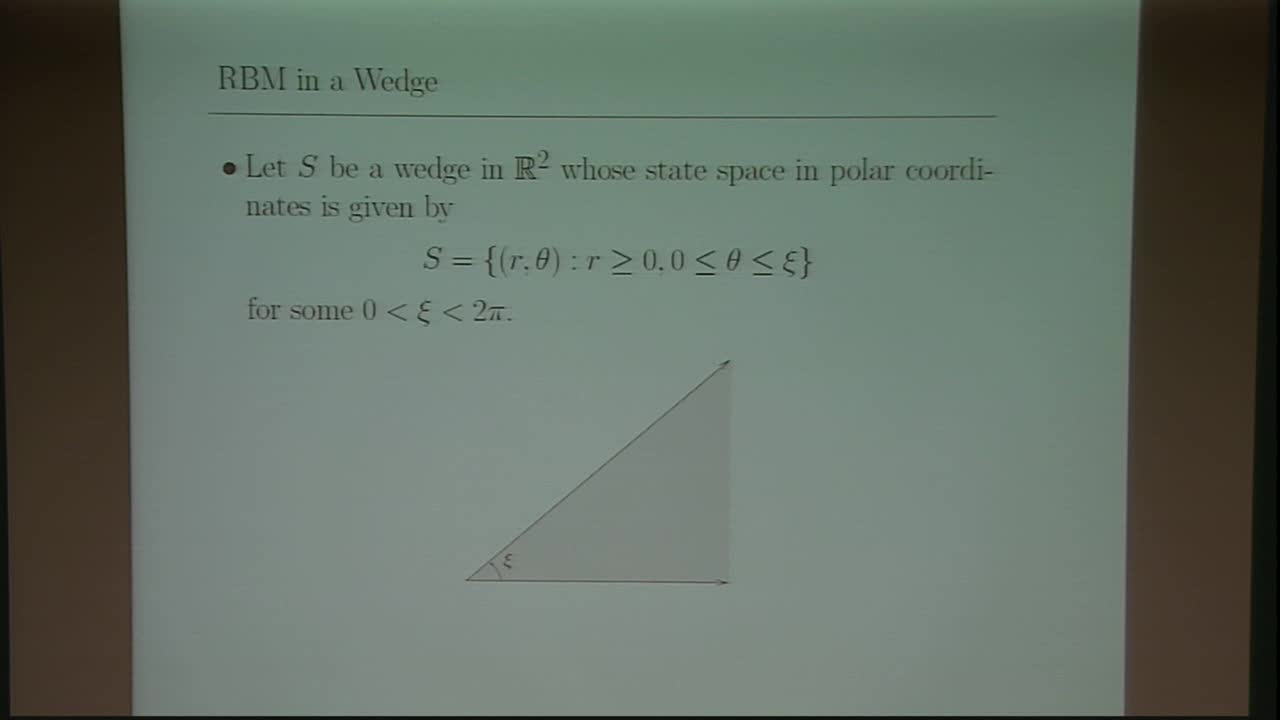## A Dirichlet Process Characterization of RBM in a Wedge

May 15, 2018
IMA
Presenters: Joshua Reed

Watch Video

#### Abstract

Reflected Brownian motion (RBM) in a wedge is a 2-dimensional stochastic process $Z$ whose state space in $\mathbb{R}^2$ is given in polar coordinates by $S=\{(r,\theta): r \geq 0, 0 \leq \theta \leq \xi\}$ for some $0 < \xi < 2 \pi$. Let $\alpha= (\theta_1+\theta_2)/\xi$, where $-\pi/2 < \theta_1,\theta_2 < \pi/2$ are the directions of reflection of $Z$ off each of the two edges of the wedge as measured from the corresponding inward facing normal. We prove that in the case of $1 < \alpha < 2$, RBM in a wedge is a Dirichlet process. Specifically, its unique Doob-Meyer type decomposition is given by $Z=X+Y$, where $X$ is a two-dimensional Brownian motion and $Y$ is a continuous process of zero energy. Furthermore, we show that for $p > \alpha$, the strong $p$-variation of the sample paths of $Y$ is finite on compact intervals, and, for $0 < p \leq \alpha$, the strong $p$-variation of $Y$ is infinite on $[0,T]$ whenever $Z$ has been started from the origin. We also show that on excursion intervals of $Z$ away from the origin, $(Z,Y)$ satisfies the standard Skorokhod problem for $X$. However, on the entire time horizon $(Z,Y)$ does not satisfy the standard Skorokhod problem for $X$, but nevertheless we show that it satisfies the extended Skorkohod problem. This is joint work with Peter Lakner and Bert Zwart.